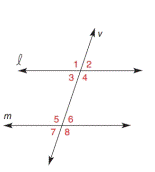Chapter 2.3, Problem 3E### Elementary Geometry for College St...

6th Edition
Daniel C. Alexander + 1 other
ISBN: 9781285195698

#### Solutions

Chapter
Section### Elementary Geometry for College St...

6th Edition
Daniel C. Alexander + 1 other
ISBN: 9781285195698
Textbook Problem
1 views

# In Exercises 1 to 6, l and m are cut by transversal v . On the basis of the information given, determine whether l must be parallel to m .m ∠ 1 = 106 ° and m ∠ 7 = 76 °

To determine

To verify:

Whether 𝓁 must be parallel to m.

Explanation

Given:

𝓁 and m are cut by transversal v.

m1=106° and m7=76°.

Figure (1)

Approach:

(1) If two lines are cut by a transversal so that two corresponding angles are congruent, these lines are parallel.

(2) The supplementary angles add up to 180°.

Calculation:

The given statement is, 𝓁 and m are cut by transversal v.

m1=106° and m7=76°

7 and 5 are supplementary angles.

So, m7+m5=180°

Substitute 76° for m7 in the above equation.

### Still sussing out bartleby?

Check out a sample textbook solution.

See a sample solution

#### The Solution to Your Study Problems

Bartleby provides explanations to thousands of textbook problems written by our experts, many with advanced degrees!

Get Started

#### Find more solutions based on key concepts